Next: The 2-d resonant cavity Up: The wave equation Previous: Upwind differencing

## The 1-d wave equation

Consider a plane polarized electromagnetic wave propagating in vacuo along the-axis. Suppose that the electric and magnetic fields take the form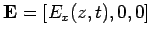, and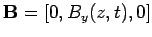. Now, according to Maxwell's equations,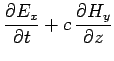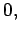(253)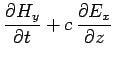(254)

where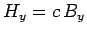, and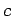is the velocity of light. Note that the above equations take the form of two coupled advection equations. Let us find the numerical solution of these equations in some region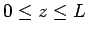which is bounded by perfectly conducting walls at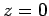and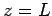. Now, both the tangential electric field and the normal magnetic field must be zero at a perfect conductor. It follows that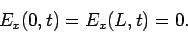(255)

Moreover, Eq. (253) yields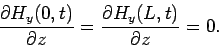(256)

We expect Eqs. (253) and (254) to support wave-like solutions which bounce backwards and forwards between the conducting walls.

As before, we discretize in time on the uniform grid, for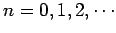. Furthermore, in the-direction, we discretize on the uniform grid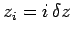, for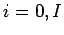, where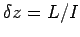. Adopting a Crank-Nicholson temporal differencing scheme similar to that discussed in Sect. 7.4, Eqs. (253)-(254) yield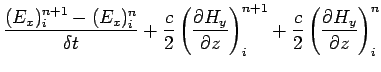(257)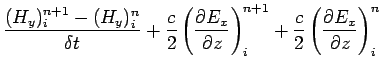(258)

where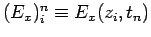, etc.

Adopting a Fourier approach, we write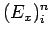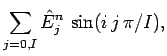(259)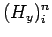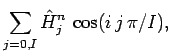(260)

which automatically satisfies the boundary conditions (255) and (256). Equations (257) and (258) yield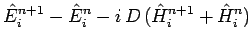(261)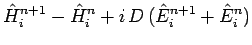(262)

where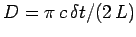. It follows that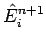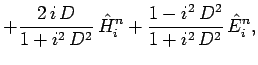(263)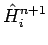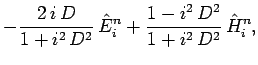(264)

for.

Let us determine the eigenvalues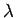of the above linear system, assuming that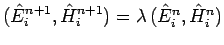. After a little analysis, we obtain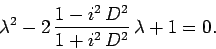(265)

For all values of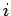, the two roots of the above quadratic are complex, but have modulus unity: i.e.,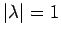. This implies that our differencing scheme is both numerically stable (if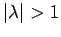then the scheme would be unstable, since the associated eigenfunction would be amplified at each time-step) and non-dispersive (if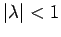then all Fourier harmonics would eventually decay away, and, hence, so would the solution). Note that the above calculation is equivalent to von Neumann stability analysis.

The routine listed below solves the 1-d wave equation using the Crank-Nicholson scheme discussed above. The routine first Fourier transforms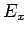and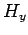, takes a time-step using Eqs. (263) and (264), and then reconstructsandvia an inverse Fourier transform.

// Wave1D.cpp

// Function to evolve 1-d wave equation:

//  d E_x / dt + c d H_y / dz = 0

//  d H_y / dt + c d E_x / dz = 0

// in region 0 < z < L.

// Boundary conditions:

//  E_x(0,t) = E_x(L,t) = 0

//  d H_y(0,t) / dz =  d H_y(L,t) / dz = 0

// Arrays Ex, Hy assumed to be of extent I+1.

// Now, ith elements of arrays correspond to

//  z_i = i * L / J      i=0,I

// Also, D = pi c dt / (2 L), where dt is time-step.

// Uses Crank-Nicholson scheme.

#include <blitz/array.h>

using namespace blitz;

void fft_forward_cos (Array<double,1> f, Array<double,1>& F);
void fft_backward_cos (Array<double,1> F, Array<double,1>& f);
void fft_forward_sin (Array<double,1> f, Array<double,1>& F);
void fft_backward_sin (Array<double,1> F, Array<double,1>& f);

void Wave1D (Array<double,1>& Ex, Array<double,1>& Hy, double D)
{
// Find I. Declare local arrays
int I = Ex.extent(0) - 1;
Array<double,1> EE(I+1), HH(I+1), EE0(I+1), HH0(I+1);

// Fourier transform Ex and Hy
fft_forward_sin (Ex, EE0);
fft_forward_cos (Hy, HH0);

// Evolve EE and HH
for (int i = 0; i <= I; i++)
{
double x = double (i) * D;
double fp = 1. + x*x;
double fm = 1. - x*x;

EE(i) = 2. * x * HH0(i) + fm * EE0(i);
EE(i) /= fp;
HH(i) = - 2. * x * EE0(i) + fm * HH0(i);
HH(i) /= fp;
}

// Reconstruct Ex and Hy via inverse Fourier transform
fft_backward_sin (EE, Ex);
fft_backward_cos (HH, Hy);
}


Figure 230 shows an example calculation which uses the above routine to propagate a Gaussian wave-pulse. The initial condition is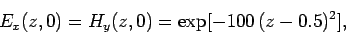(266)

and the calculation is performed with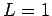,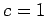,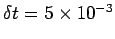, and. It can be seen that the pulse moves to the right, reflects off the conducting wall at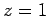, and then moves to the left. Note, that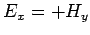when the wave is far from the conducting walls and moving to the right, whereas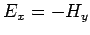when the wave is far from the conducting walls and moving to the left.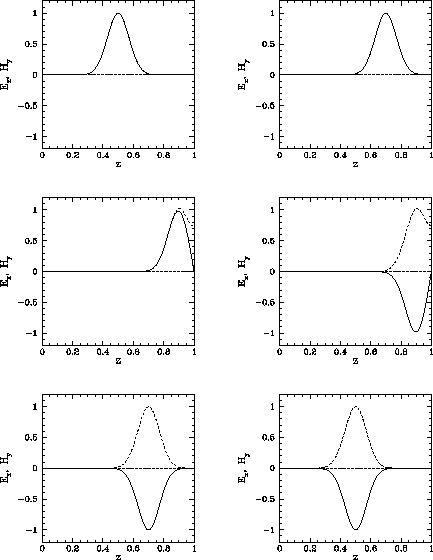Figure 253 shows a second example calculation performed with the same parameters as the first. In this calculation, the pulse is allowed to reflect off the conducting walls ten times before returning to its initial position. Note that the pulse amplitude and shape remain constant to a very good approximation during this process. The fact that the pulse returns almost exactly to its initial position after ten time periods have elapsed (i.e., at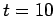) demonstrates that it is propagating at the correct speed (i.e.,).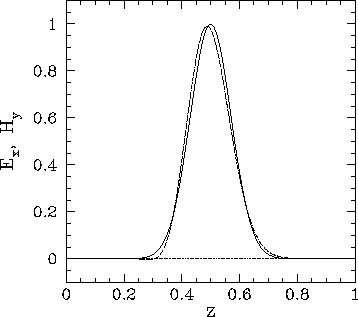Figure 82 shows a third example calculation which uses the above listed routine to propagate a square wave-pulse. Note that the routine does a very poor job, since spurious oscillations are generated at the sharp leading and trailing edges of the wave-form. As discussed in Sect. 7.5, such oscillations can only be suppressed by adopting an upwind differencing scheme, which in this case means that the spatial differences must be skewed in the direction from which the wave is propagating. Unfortunately, simple explicit upwind schemes are subject to the CFL constraint,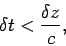(267)

and also tend to be highly dispersive.Next: The 2-d resonant cavity Up: The wave equation Previous: Upwind differencing
Richard Fitzpatrick 2006-03-29